## Percentage For SBI PO : Set -09

1) Ram sells a book at a profit of 5%.If he had bought it at 10% less and sold it for Rs.6 more, he would have gained 20%. Find the cost price of the book?

a) Rs.200

b) Rs.300

c) Rs.400

d) Rs.250

e) Rs.240

a)

Let the Cost Price of book be Rs.9

initial S.P = Rs.1.05P

New C.P = Rs.0.90P

New C.P = 1.20*0.90P

P=1.08

1.08P-1.05P=6

0.03P=6

p=Rs.200

2) The price of sugar is increased by 28%. Therefore a family reduces its consumption so that the increment in the expenditure of sugar is only 20%. If the consumption of sugar is 15 kg before the increment, what is the consumption now?

a) 15 1/16 kg

b) 14 1/16 kg

c) 14 3/16 kg

d) 14 1/18 kg

e) 15 1/8 kg

(b)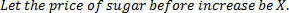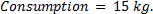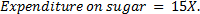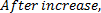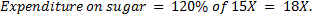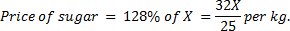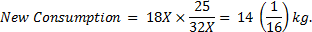3) Ms. Sujata invests 7% i.e. Rs.2170 of her monthly salary in mutual funds. Later she invests 18% of her monthly salary in recurring deposits also, she invests 6% of her salary on NSC’s. What is the total annual amount invested by Ms. Sujata?

a) 1,25,320

b) 1,13,520

c) 1,35,120

d) 1,15,320

e) none of these

d)

let her monthly salary be rs. x

According to the Question,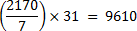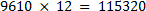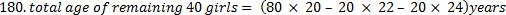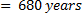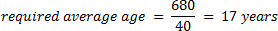4) A person could save 10% of his income. But 2 years later, when his income increased by 20%, he could save the same amount only as  before. By how much percentage has his expenditure increased?

a) 22 2/9%

b) 23 1/3%

c) 24 2/9%

d) 25 2/9%

e) none of these

a)

Income be 100 = 10% = rs.10 Saving

90% = rs.90 Expenditure

2 years later, income increased by 20%

=(100*120/100)=Rs.120

2 years later income increased by 20%

According to questions, saving same amount of Rs.10 after 2 years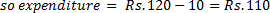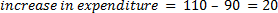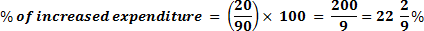5) In an examination, Raman scored 25 marks less than Rohit. Rohit scored 45 more marks than Sonia. Rohan scored 75 marks which is 10 more than Sonia. Ravi’s score is 50 less than, maximum marks of the test. What approximate percentage of marks did Ravi score in the examination, if he gets 34 marks more than Raman?

a) 90

b) 70

c) 80

d) 60

e) 85

b)

according to questions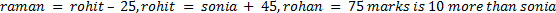So sonia mark = 65

Rohit = 110(65+45)

Raman = 110-25=85

Ravi = 85+34= 119

Total Mark = 119 +50 -169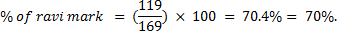6) If A’s income is 25% higher than B’s income, then how much percent is B’s income lower than A’s income?

a) 25

b) 30

c) 35

d) 20

e) None of these

d)

Let the income of B is 100, then A’s income =125;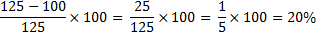7) In a class, 40% of the boys is same as 1/2 of the girls and there are 20 girls. Total number of students in the class is:

a) 70

b) 45

c) 35

d) 25

e) None of these

b)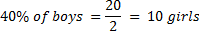Total number of boys = 25

Total number of students = 25 + 20 = 45

8) In a class 50% of students passed in French, 60% 0f students passed in English. Then if every student passed in either French or English or both the subjects.Then what is the  percentage of students passed In both the subjects?

a) 10%

b) 20%

c) 15%

d) 12%

e) 25%

a)

n (F)=50%

n (E)=60%

n (F)+n(E) -n(F U E)=n(F n E)
60+50 -100  = 10 %

9) A number X is increased by 20% to get another number Y. A third number Z is obtained by increasing Y by 10%. By what per cent should Z be decreased to get X?

a) 26.51%

b) 24.24%

c) 28.36%

d) 31.71%

e) 34.98%

b)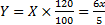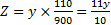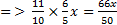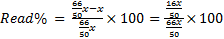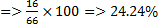10) When the prices of cloth were reduced by 25% the quantity of cloth sold increased by 20%. What was the effect on gross receipt of the shop?

a) 5% increase

b) 5% decrease

c) No change

d) 10% increase

e) None of these